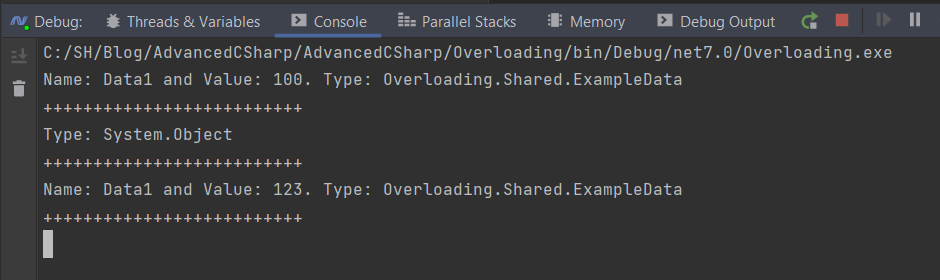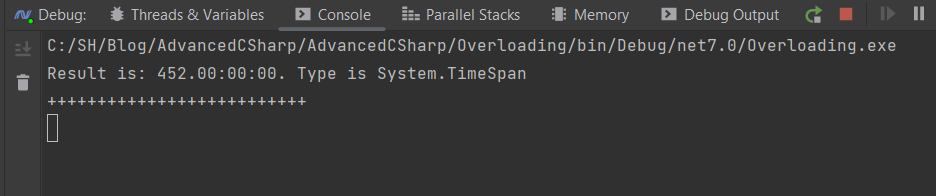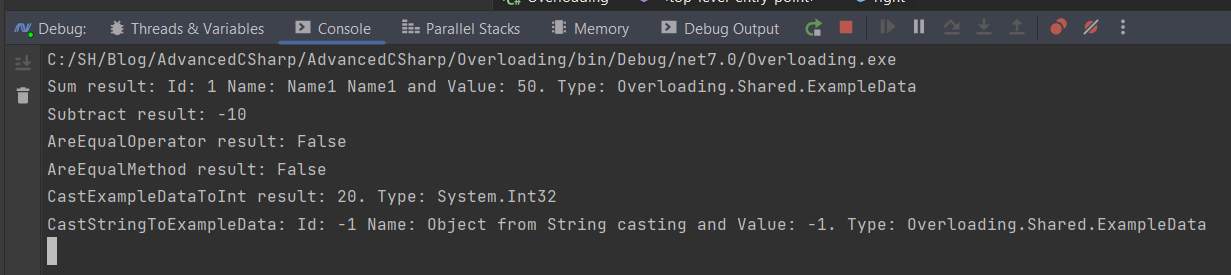BLOG
22 January 2023

tech

In C#, there might be two or more methods in a class with the same name but different numbers, types, and order of parameters - it is called method overloading. This is what the definition says, and that is 100% correct. But the description does not state that methods are not the only element that can be overloaded. Plus, the overloading technic can be used in other cases. So, the definition, as it often is, is just the tip of the iceberg. Let’s see what hides out there.

If you want to download the code, you can find it at GitHub. The project names match the blog post topic.

Before we dive into actual overloading, we need to make some preparations. For the project we will be using a simple console application, so nothing fancy. The project also needs to have some classes we will use in our examples. In this case, the class name is ExampleData.cs. The class is very simple. At least for now.

``````1
2
3
4
5
6
7
8
``````namespace Overloading.Shared;

public class ExampleData
{
public int Id { get; set; }
public int Value { get; set; }
public string Name { get; set; }
} ``````

The actual coding starts now. The first thing is to create a class to hold method overloading examples. The class name is MethodExample.cs and the code inside the class looks like this.

``````1
2
3
4
5
6
7
8
9
10
11
12
13
14
15
16
17
18
19
20
21
22
``````using Overloading.Shared;

public class MethodExample
{
public string ProcessData(ExampleData exampleData)
{
return \$"Name: {exampleData.Name} and Value: {exampleData.Value}. Type: {exampleData.GetType()}";
}
public string ProcessData(object exampleData)
{
return \$"Type: {exampleData.GetType()}";

}

public string ProcessData(ExampleData exampleData, int changeValueBy)
{
return \$"Name: {exampleData.Name} and Value: {exampleData.Value + changeValueBy}. Type: {exampleData.GetType()}";
}
} ``````

As we can see, the class has three methods, all of which have the same name. The fact that they have different input settings is what interests us right now - that is method overloading for you. When using the ProcessData method, the input must now match one of the expected options. Sounds easy and in most cases it is, but still, we need to see the code in action to be certain we understand it. The sample code is in the Program.cs file.

``````1
2
3
4
5
6
7
8
9
10
11
12
13
14
15
16
17
18
``````using Overloading.MethodsExample;

var methodExample = new MethodExample();
var exampleData = new ExampleData()
{
Id = 1,
Name = "Data1",
Value = 100

};
var exampleObject = new object();
Console.WriteLine(methodExample.ProcessData(exampleData));
Console.WriteLine("++++++++++++++++++++++++++");
Console.WriteLine(methodExample.ProcessData(exampleObject));
Console.WriteLine("++++++++++++++++++++++++++");
Console.WriteLine(methodExample.ProcessData(exampleData, 23));
Console.WriteLine("++++++++++++++++++++++++++"); ``````

The code shows the examples of calling the method with a set of parameters. In two out of three examples it works exactly like you might have expected. The most interesting example is the second one, in which we are passing an object of type object, and the runtime is smart enough to match the right approach. As it can be seen in the code execution result screen below.Now that the easy part is behind us, time for something more interesting.

Why?

I’m going to give you a piece of code and, using it, work my way towards the goal I’m trying to make.

``````1
2
3
4
5
``````var startDate = new DateTime(2020, 10, 6);
var endDate = new DateTime(2022, 1, 1);
var span = endDate - startDate;
Console.WriteLine(\$"Result is: {span}. Type is {span.GetType()}");
Console.WriteLine("++++++++++++++++++++++++++"); ``````

In this code, we are doing something strange at first glance. We are subtracting a date from a date and strangely enough, it works, as you can see below.The result is the time span between these two dates. In this case it is 452 days. But how does it work? When we think about subtracting we think numbers not dates. The answer lies in the guts of DataTime type definition. Inside the DateTime.cs file we can find this gem.

``````1
2
3
4
5
6
7
8
``````public static DateTime operator -(DateTime d, TimeSpan t)
{
ulong ticks = (ulong)(d.Ticks - t._ticks);
if (ticks > MaxTicks) ThrowDateArithmetic(1);
return new DateTime(ticks | d.InternalKind);
}

public static TimeSpan operator -(DateTime d1, DateTime d2) => new TimeSpan(d1.Ticks - d2.Ticks); ``````

This gem is two subtract operator overloads. Yes, we can overload the operator. By doing so, we can modify the behaviour of an already defined operator or add an operator to a type where there wasn’t one. This gives us a huge range of possibilities to shape the behaviour of type to our needs. As we already know that operator overloading is an option, the time has come to modify our ExampleData class.

Our ExampleData class needs to be changed quite a lot for it to override operators. So now the class looks like this.

``````1
2
3
4
5
6
7
8
9
10
11
12
13
14
15
16
17
18
19
20
21
22
23
24
25
26
27
28
29
30
31
32
33
34
35
36
37
38
39
40
41
42
43
44
45
46
47
48
49
50
51
52
53
54
55
56
57
58
59
60
61
62
63
64
65
66
67
68
69
70
71
72
73
74
75
76
77
78
79
80
81
82
83
84
85
86
87
88
89
90
91
92
93
94
95
96
97
98
99
100
101
102
103
104
105
106
107
108
109
``````namespace Overloading.Shared;

public class ExampleData : IEquatable<ExampleData>, IComparable<ExampleData>, IComparable

{
public int CompareTo(ExampleData? other)
{
if (ReferenceEquals(this, other)) return 0;
if (ReferenceEquals(null, other)) return 1;
return Value.CompareTo(other.Value);
}

public int CompareTo(object? obj)
{
if (ReferenceEquals(null, obj)) return 1;
if (ReferenceEquals(this, obj)) return 0;
return obj is ExampleData other ? CompareTo(other) : throw new ArgumentException(\$"Object must be of type {nameof(ExampleData)}");

}
public static bool operator <(ExampleData? left, ExampleData? right)
{
return Comparer<ExampleData>.Default.Compare(left, right) < 0;
}
public static bool operator >(ExampleData? left, ExampleData? right)
{
return Comparer<ExampleData>.Default.Compare(left, right) > 0;
}

public static bool operator <=(ExampleData? left, ExampleData? right)
{
return Comparer<ExampleData>.Default.Compare(left, right) <= 0;
}

public static bool operator >=(ExampleData? left, ExampleData? right)
{
return Comparer<ExampleData>.Default.Compare(left, right) >= 0;
}
public bool Equals(ExampleData? other)
{
if (ReferenceEquals(null, other)) return false;
if (ReferenceEquals(this, other)) return true;
return Id == other.Id && Value == other.Value && Name == other.Name;
}

public override bool Equals(object? obj)
{
if (ReferenceEquals(null, obj)) return false;
if (ReferenceEquals(this, obj)) return true;
if (obj.GetType() != this.GetType()) return false;
return Equals((ExampleData)obj);
}
public override int GetHashCode()
{
return HashCode.Combine(Id, Value, Name);
}
public static bool operator ==(ExampleData? left, ExampleData? right)
{
return Equals(left, right);
}

public static bool operator !=(ExampleData? left, ExampleData? right)
{
return !Equals(left, right);
}

public static ExampleData operator +(ExampleData? left, ExampleData? right)
{
if (ReferenceEquals(null, left))
left = new ExampleData();
if (ReferenceEquals(null, right))
right = new ExampleData();
return new ExampleData()

{
Id = left.Id,
Value = left.Value + right.Value,
Name = left.Name + " " + right.Name
};
}

public static int operator -(ExampleData? left, ExampleData? right)
{
if (ReferenceEquals(null, left))
left = new ExampleData();
if (ReferenceEquals(null, right))
right = new ExampleData();
return left.Value - right.Value;
}

public static implicit operator int(ExampleData exampleData)
{
return exampleData.Value;
}

public static explicit operator ExampleData(string name)
{
return new()
{
Id = -1,
Value = -1,
Name = name
};
}

public int Id { get; set; }
public int Value { get; set; }
public string Name { get; set; }
} ``````

I know you may be surprised by the length of this class but bear with me.

There are three distinct areas that need our attention. First is dealing with equality, which is tricky, because you are required to provide all the equalities and their combinations (!=, >=, > …) implementation. This is forced on you by the interfaces you need to implement. This code was generated by the Rider. I strongly encourage you not to implement it yourself. But to use the tool and modify the result to your needs. In this case, I didn’t modify anything related to the equality operator. I want to show you that you can do it and encourage you to debug the process of comparing two ExampleData objects.

The second part holds two custom operator overrides - as you can see, we have overloaded adding and subtracting. The interesting thing about adding is how two objects are merged into one. In subtract overload, the thing that should draw our attention is that it returns an int that results in subtracting the value property of both objects.

The third and final part is casting overloading. Yes, we can do that. In this example, we have defined two custom castings: one is an implicit casting that casts ExampleData object to int, and the other is an explicit one. This casting allows us to cast string to ExampleData object.

These overloaded actions are being used by OperatorsExample class.

``````1
2
3
4
5
6
7
8
9
10
11
12
13
14
15
16
17
18
19
20
21
22
23
24
25
26
27
28
29
30
31
32
33
34
35
36
37
38
39
``````using Overloading.Shared;

public class OperatorsExample
{
public bool AreEqualOperator(ExampleData left, ExampleData right)
{
return left == right;
}

public bool AreEqualMethod(ExampleData left, ExampleData right)
{
return left.Equals(right);
}
public ExampleData Add(ExampleData left, ExampleData right)
{
return left + right;
}

public int Substract(ExampleData left, ExampleData right)
{
return left - right;
}

public int CastExampleDataToInt(ExampleData exampleData)
{
int result = exampleData;
return result;
}

public ExampleData CastStringToExampleData(string name)
{
var result = (ExampleData)name;
return result;
}
} ``````

Now with that class in place we can use these overloaded methods in our code. The Program.cs class code looks like so.

``````1
2
3
4
5
6
7
8
9
10
11
12
13
14
15
16
17
18
19
20
21
22
``````var operators = new OperatorsExample();
var left = new ExampleData()
{
Id = 1,
Name = "Name1",
Value = 20
};
var right = new ExampleData()
{
Id = 2,
Name = "Name1",
Value = 30
};
Console.WriteLine(\$"Sum result: Id: {sumResult.Id} Name: {sumResult.Name} and Value: {sumResult.Value}. Type: {sumResult.GetType()}");
Console.WriteLine(\$"Subtract result: {operators.Substract(left, right)}");
Console.WriteLine(\$"AreEqualOperator result: {operators.AreEqualOperator(left,right)}");
Console.WriteLine(\$"AreEqualMethod result: {operators.AreEqualMethod(left,right)}");
var toIntResult = operators.CastExampleDataToInt(left);
Console.WriteLine(\$"CastExampleDataToInt result: {toIntResult}. Type: {toIntResult.GetType()}");
var fromStringResult = operators.CastStringToExampleData("Object from String casting");
Console.WriteLine(\$"CastStringToExampleData: Id: {fromStringResult.Id} Name: {fromStringResult.Name} and Value: {fromStringResult.Value}. Type: {fromStringResult.GetType()}"); ``````

Running above code will give us the results we expect. I strongly encourage you, again, to debug the entire process and analyse how it works in details.## Summary

This was the third article in the Advanced C# series. We've talked about overloading in a few different contexts. Additionally, we have seen how this method makes code more flexible towards our needs. Next time, we will expand the knowledge gained by working with extension methods.

If you have any questions, please drop me a line at karol.rogowski@softwarehut.com

Till next time, keep coding.Karol Rogowski